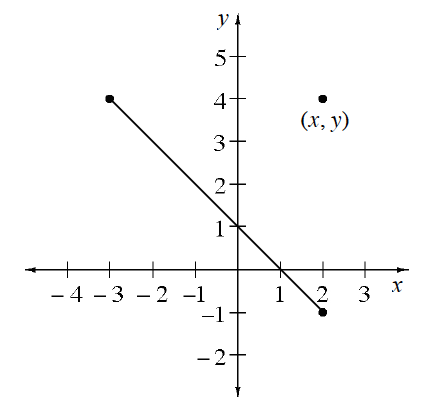### Home > CCA2 > Chapter C > Lesson C.1.1 > ProblemC-9

C-9.

In the diagram at right, the point $(x, y)$ is the same distance from $(-3, 4)$ as it is from $(2, -1)$. There are many possibilities for $(x, y)$. Algebraically, what do they have in common?

1. What is the distance from $(x, y)$ to $(-3, 4)$?

Do you remember the equation to determine the distance between two points?

$\sqrt{(x+3)^{2}+(y-4)^{2}}$

2. What is the distance from $(x, y)$ to $(2, -1)$?

Use the same equation as in part (a).

3. Write the equation that states that the two expressions in (a) and (b) are equal and simplify.

Set your two distance equations from parts (a) and (b) equal to each other.
Then simplify this larger equation until it is in $y = mx + b$ form.

$y = x + 2$

4. What is the specific name of the geometric object represented by your equation from part (c)?

Try graphing your answer from part (c).
How does this new graph relate to the original line?